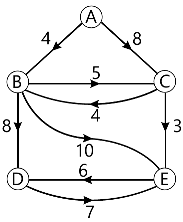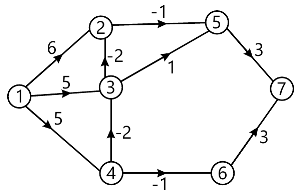MORE IN Analysis of Algorithms
MU Computer Engineering (Semester 4)
Analysis of Algorithms
May 2013
Total marks: --
Total time: --
INSTRUCTIONS
(1) Assume appropriate data and state your reasons
(2) Marks are given to the right of every question
(3) Draw neat diagrams wherever necessary

1 (a) Explain Divide and Conquer strategy. Write control abstraction (general method) for it. List any four examples that can be solved by divide and conquer.
10 M
1 (b) Explain asymptotic notations. Explain time complexity and space complexity in detail.
10 M

2 (a) Explain Graph Colouring problem using backtracking. Write algorithm for same.
10 M
2 (b) Find out single source shortest path for following problem using Dijkstra's Algorithm.10 M

3 (a) Find the longest common subsequence from the given two sub-sequences:-
P = {100101101101}
Q = {0110}
10 M
3 (b) Explain 15 puzzle problem using Branch and Bound.
10 M

4 (a) Sort following numbers using Quicksort algorithm.
65, 70, 75, 80, 85, 60, 55, 50, 45.
Show all passes of execution. Also state the time complexity.
10 M
4 (b) Explain and write Knuth-Morris Pratt algorithm. Explain with an example.
10 M

5 (a) Explain job sequencing with deadlines. Solve the following instance: n=5
(P1, P2, P3, P4, P5) = (20, 15, 10, 5, 1)(d1, d2, d3, d4, d5) = (2, 2, 1, 3, 3)
10 M
5 (b) Solve the following sum of subset problem using backtracking:
w= {1, 3, 4, 5}, m=8.
Find the possible subsets of 'w' that sum to 'm'.
10 M

6 (a) Solve shortest path from source 1 for following graph using dynamic programming.10 M
6 (b) Explain travelling Salesperson Problem using branch and bound method.
10 M

Write short notes:-
7 (a) Differentiate between greedy approach and dynamic programming.
5 M
7 (b) Optimal Storage On Tapes
5 M